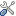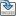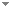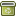# 2. Programming relativity as the mathematics of perspective in a Planck unit Simulation Hypothesis

 Authors Abstract The Simulation Hypothesis proposes that all of reality is in fact an artificial simulation, analogous to a computer simulation. Outlined here is a method for programming relativistic mass, space and time at the Planck level as applicable for use in Planck Universe-as-a-Simulation Hypothesis. For the virtual universe the model uses a 4-axis hyper-sphere that expands in incremental steps (the simulation clock-rate). Virtual particles that oscillate between an electric wave-state and a mass point-state are mapped within this hyper-sphere, the oscillation driven by this expansion. Particles are assigned an N-S axis which determines the direction in which they are pulled along by the expansion, thus an independent particle motion may be dispensed with. Only in the mass point-state do particles have fixed hyper-sphere co-ordinates. The rate of expansion translates to the speed of light and so in terms of the hyper-sphere co-ordinates all particles (and objects) travel at the speed of light, time (as the clock-rate) and velocity (as the rate of expansion) are therefore constant, however photons, as the means of information exchange, are restricted to lateral movement across the hyper-sphere thus giving the appearance of a 3-D space. Lorentz formulas are used to translate between this 3-D space and the hyper-sphere co-ordinates, relativity resembling the mathematics of perspective. Keywords black-hole  relativity  hyper-sphere  simulation hypothesis  mathematics of perspective  mathematical universe  Planck unit  programming Categories (categorize this paper) OptionsEdit this recordMark as duplicateExport citationFind it on ScholarRequest removal from indexRevision history

PhilArchive copy

Setup an account with your affiliations in order to access resources via your University's proxy server
Configure custom proxy (use this if your affiliation does not provide a proxy)

## References found in this work BETA

.Nick Bostrom & Julian Savulescu - 2009 - Oxford University Press.

## Citations of this work BETA

No citations found.